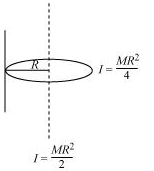## Pages

### System of particles and Rotational motion NCERT Solutions Class 11 Physics - Solved Exercise Question 7.10

Question 7.10:
(a) Find the moment of inertia of a sphere about a tangent to the sphere, given the moment of inertia of the sphere about any of its diameters to be 2MR2/5, where M is the mass of the sphere and R is the radius of the sphere.
(b) Given the moment of inertia of a disc of mass M and radius R about any of its diameters to be MR2/4, find its moment of inertia about an axis normal to the disc and passing through a point on its edge.
Solution:
(a) The moment of inertia (M.I.) of a sphere about its diameter = 2MR2/5According to the theorem of parallel axes, the moment of inertia of a body about any axis is equal to the sum of the moment of inertia of the body about a parallel axis passing through its centre of mass and the product of its mass and the square of the distance between the two parallel axes.
The M.I. about a tangent of the sphere = 2MR2/5 + MR =  7MR2 / 5

(b) The moment of inertia of a disc about its diameter = MR2 / 4
According to the theorem of perpendicular axis, the moment of inertia of a planar body (lamina) about an axis perpendicular to its plane is equal to the sum of its moments of inertia about two perpendicular axes concurrent with perpendicular axis and lying in the plane of the body.
The M.I. of the disc about its centre = MR2 / 4 + MR2 / 4  =  MR2 / 2
The situation is shown in the given figure.Applying the theorem of parallel axes:
The moment of inertia about an axis normal to the disc and passing through a point on its edge
= MR2 / 2 + MR2 = 3MR2 / 2